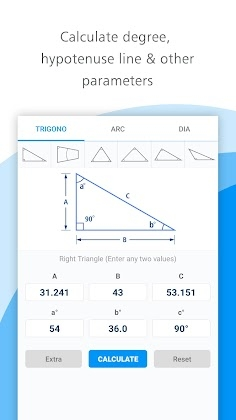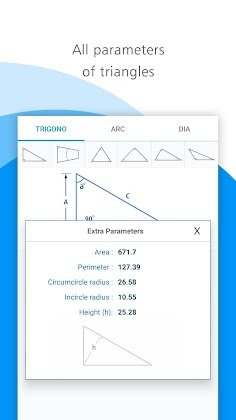Triangle Calculator & Taper Degree Calculator Mod Apk 3.2 Premium latest

Name Triangle Calculator & Taper Degree Calculator Mod Apk 3.2 Premium latest 12 Sep 2021 Apps > Tools Premium - Subscription Unlocked 5.0 and up Aptimo com.aptimo.techno.anglecal 6.50 MB

Triangle Calculator & Taper Degree Calculator 3.2 Apk Premium latest is a Tools Android app

Download Triangle Calculator & Taper Degree Calculator Apk Premium and enjoy one the fantastic Tools app for Android.

Easy triangle calculator application for finding degree, taper and hypotenuse length with additional options such as Arc radius, angle, cord length, cord height and area and circumcircle dia. and incircle dia. of the geometrical shapes.Find out degree and sides by entering two values for Right triangle and three values for triangle, this triangle calculator app also provides you many parameter like, Side length, degree, Area, Perimeter, Circumcircle radius, Incircle radius and Height

Types of triangle
* Right triangle
* Taper Degree
* Triangle
* Isosceles triangle
* Scalene triangle
* Obtuse triangle

Arc Calculation
* Arc degree
* Cord length
* Cord height
* Segment area
* Sector area.

Circumcircle diameter and incircle diameter of the geometrical shapes (diameter of the workpiece)
* Equal Triangle
* Square
* Rectangle
* Pentagon
* Hexagon
* OctagonVery useful for machining purpose, easy to use and quick results.

What’s New:
Arc and diameter of the geometrical shapes option added.

Subscription Unlocked